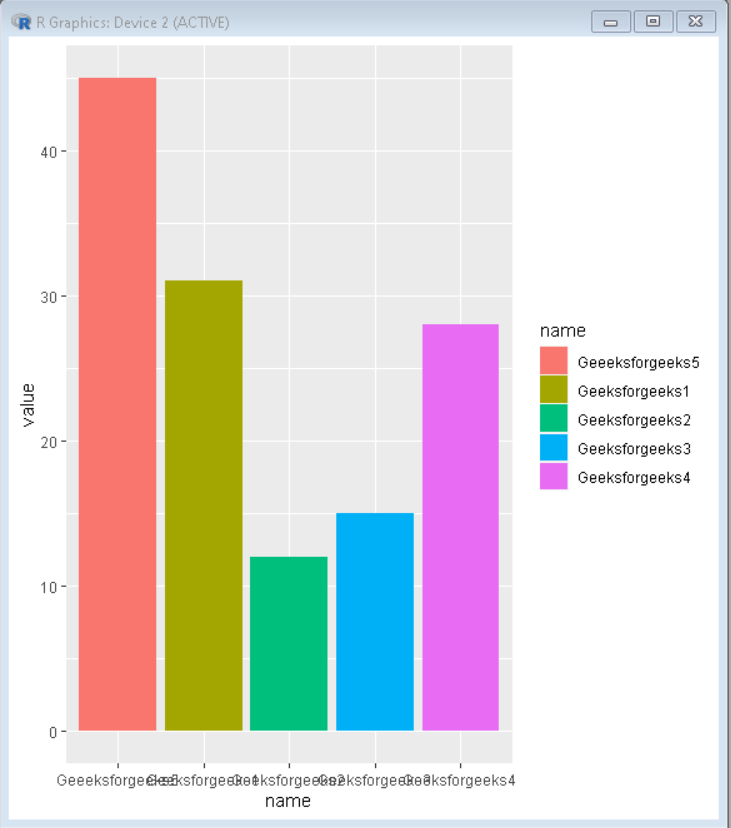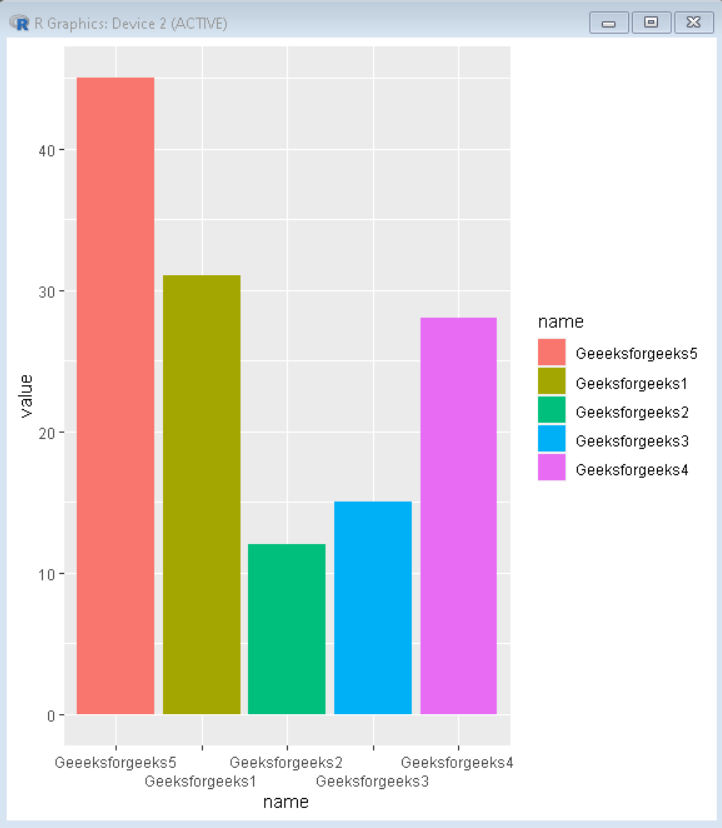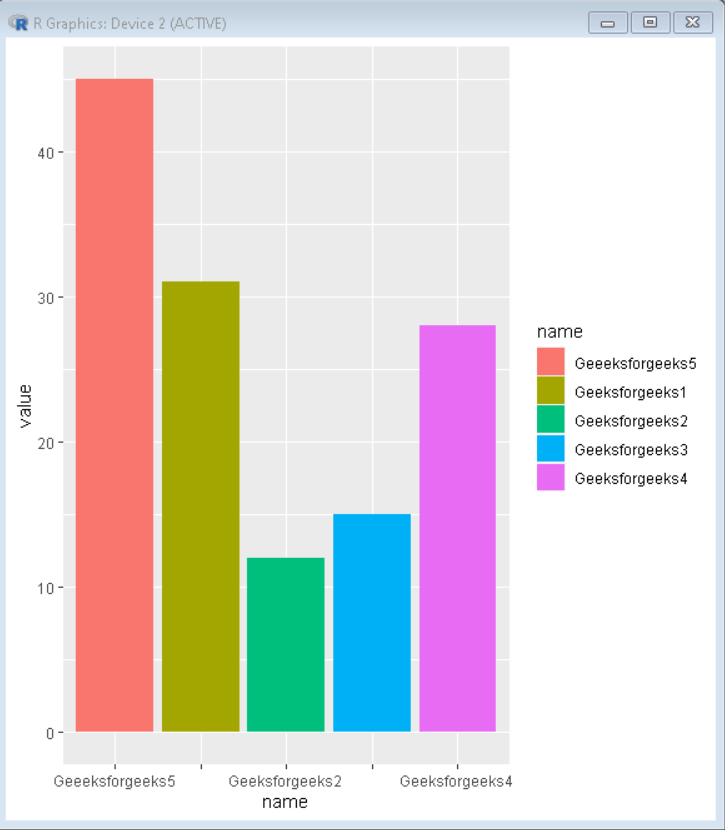GeeksforGeeks App
Open AppBrowser
Continue

# How to Avoid Overlapping Labels in ggplot2 in R?

In this article, we are going to see how to avoid overlapping labels in ggplot2 in R Programming Language.

To avoid overlapping labels in ggplot2, we use guide_axis() within scale_x_discrete().

Syntax: plot+scale_x_discrete(guide = guide_axis(<type>))

In the place of we can use the following properties:

• n.dodge: It makes overlapping labels shift a step-down.
• check.overlap: This removes the overlapping labels and displays only those which do not overlap

## R

 `# Create sample data``set.seed``(5642)                            ``sample_data <- ``data.frame``(name = ``c``(``"Geeksforgeeks1"``,``                                   ``"Geeksforgeeks2"``,``                                   ``"Geeksforgeeks3"``,``                                   ``"Geeksforgeeks4"``,``                                   ``"Geeeksforgeeks5"``) ,``                          ``value = ``c``(31,12,15,28,45))``# Load ggplot2 package``library``(``"ggplot2"``)` `# Create bar plot``plot<-``ggplot``(sample_data, ``aes``(name,value, fill=name)) +``geom_bar``(stat = ``"identity"``)``plot`

Output:## Avoid overlapping labels

To avoid overlapping by shifting labels downward we use n.dodge parameter of guide_axis() function:

## R

 `# Create sample data``set.seed``(5642)                            ``sample_data <- ``data.frame``(name = ``c``(``"Geeksforgeeks1"``,``                                   ``"Geeksforgeeks2"``,``                                   ``"Geeksforgeeks3"``,``                                   ``"Geeksforgeeks4"``,``                                   ``"Geeeksforgeeks5"``) ,``                          ``value = ``c``(31,12,15,28,45))``# Load ggplot2 package``library``(``"ggplot2"``)` `# Create bar plot without overlapping labels``plot<-``ggplot``(sample_data, ``aes``(name,value, fill=name)) +``geom_bar``(stat = ``"identity"``) +``scale_x_discrete``(guide = ``guide_axis``(n.dodge=2))``plot`

Output:## Remove overlapping

To remove overlapping labels we use check.overlap parameter of guide_axis() function:

## R

 `# Create sample data``set.seed``(5642)                            ``sample_data <- ``data.frame``(name = ``c``(``"Geeksforgeeks1"``,``                                   ``"Geeksforgeeks2"``,``                                   ``"Geeksforgeeks3"``,``                                   ``"Geeksforgeeks4"``,``                                   ``"Geeeksforgeeks5"``) ,``                          ``value = ``c``(31,12,15,28,45))``# Load ggplot2 package``library``(``"ggplot2"``)` `# Create bar plot without overlapping labels``plot<-``ggplot``(sample_data, ``aes``(name,value, fill=name)) +``geom_bar``(stat = ``"identity"``) +``scale_x_discrete``(guide = ``guide_axis``(check.overlap = ``TRUE``))``plot`

Output:My Personal Notes arrow_drop_up